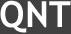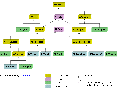home | exlaf77 | download | contact
 ExLAF77 :: Questions and Answers

How do you guarantee your implementation's accuracy?

The algorithms of evaluating transcendental functions are based on mathematically rigorous analysis of all kinds of numerical errors including iterative, truncation and round-off errors. They guarantee that the final result has NBIT correct bits of mantissa for both real and complex parts, where NBIT is a user-specified parameter (please see the documentation). In order to make sure that ExLAF77 actually provides that feature for any input values of arguments, a set of massive thorough tests has been performed including systematic comparison of results computed with different precision, and comparison with respective calculations by an independent multiprecision library. During test phase, each output result is treated as a correct one only if it appeares to be valid up to the NBIT-th bit of significand. Particular attention is paid to verification of resulting accuracy for big values of arguments and in the vicinity of singular points.

If you need guaranteed resulting accuracy of vector-matrix operations, this is easily achieved by means of specific organization of the calling program. You arrange a loop of repeated computations with incrementally increasing lengths of floating point number representations until resulting values converge to the desired accuracy.

Is ExLAF77 object-oriented?

It is object-oriented because it provides a whole hierarchy of object types with functionality ("methods") inherited down the tree. For example, any operation that can be done with a square matrix, such as calculating LU decomposition, is automatically available for Hermitian matrix (which is derived from square matrix).Click on this thumbnail to view the hierarchy chart for numbers (the link will open in a new window). Hierarchies for linear algebra objects, as well as detailed explanation of these charts, can be found in User's Manual (pp. 10 - 24).

What object types are available?

• signed and unsigned infinities;
• short (4-byte) and arbitrarily long signed Integer numbers;
• arbitrarily long signed rational numbers;
• single (4-byte) and double (8-byte) precision real and complex floating-point numbers;
• arbitrarily long real and complex floating-point numbers;
• dense real and complex vectors represented as uniform arrays of single or double precision floating-point numbers;
• dense real and complex vectors represented as uniform arrays of arbitrarily long floating-point numbers;
• dense real and complex square and rectangular matrices represented as uniform arrays of floating-point numbers of the same kinds;
• dense real and complex Hermitian matrices represented as uniform arrays of floating-point numbers of the same kinds and stored in a packed form;
• complete triangular decompositions of general real and complex square matrices in the same representations;
• complete triangular decompositions of real and complex positive-definite and indefinite Hermitian matrices in the same representations;
• Hessenberg forms of general real and complex square matrices in the same representations;
• tridiagonal forms of real and complex positive-definite and indefinite Hermitian matrices in the same representations.

Is binary input/output available?

Yes. ExLAF77 data objects can be written as binary stream to any file (e.g. on disk) and read later. These objects retain their validity also in another session. It is possible because ExLAF77 objects are stricly self-contained and location-independent.

What application languages are supported?

The library is primarily intended to be called from Fortran-77 applications (and Fortran-90/95 that include 77 standard). In particular, most routines provide alternative return label arguments. In addition, C interface for ExLAF77 is available, with Fortran's alternative return feature replaced by returning completion status.

How functionality of ExLAF77 compares to the GNUmp library?

GNUmp imposes no limit on size of mantissa / integer besides computer's physical memory, which allows GNUmp to be used for problems of computational number theory. In ExLAF77, the maximum size of mantissa is 231 bits (or 256 Megabytes). The limit on size of exponent in floating-point numbers in ExLAF77 is 231 bits (or 256 Megabytes), and in GNUmp it is one machine word (i.e. 4 or 8 bytes).

Unlike GNUmp, ExLAF77 is object-oriented in nature, so you always program for as generic types as possible.

What will be implemented next?

• increased performance of most operations;
• more operations with floating point numbers;
• more operations with generic numbers;
• more operations with matrices
• polynomial one-argument functions

 Try ExLAF77 now ExLAF77 User's Manual Demo examples
 home | exlaf77 | download | contact
© 2002 QNT Software Dev. Inc.